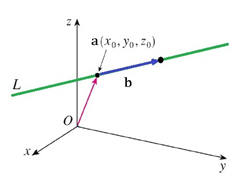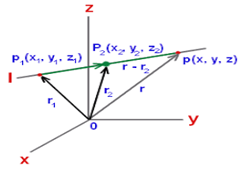#### A line with direction cosines proportional to 2, 1, 2 meets each of the linesandThe co­ordinates of each of the points of intersection are given by Option 1)Option 2)Option 3)Option 4)As we learnt in

Direction Cosines -

i)ii)    If OP =r then the co-ordinates of P will be (lr,mr,nr)

iii)    Direction cosines of X-axis are (1,0,0)

iv)    Direction cosines of Y-axis are (0,1,0)

v)    Direction cosines of Z-axis are (0,0,1)

-

Cartesian eqution of a line -

The equation of a line passing through two pointsand parallel to vector having direction ratios asis given byThe equation of a line passing through two pointsis given by- whereinPoint isNow DCs are proportional to (2, 1, 2)

SoOn solvingWe get points (3a,2a,3a) and (a,a,a).

Option 1)Incorrect Option

Option 2)Correct Option

Option 3)Incorrect Option

Option 4)Incorrect Option

#### prateek